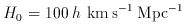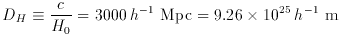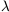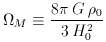### 2. COSMOGRAPHIC PARAMETERS

The Hubble constant H0 is the constant of proportionality between recession speed v and distance d in the expanding Universe;(1)

The subscripted ``0'' refers to the present epoch because in general H changes with time. The dimensions of H0 are inverse time, but it is usually written(2)

where h is a dimensionless number parameterizing our ignorance. The inverse of the Hubble constant is the Hubble time tH(3)

and the speed of light c times the Hubble time is the Hubble distance DH(4)

These quantities set the scale of the Universe, and often cosmologists work in geometric units with c = tH = DH = 1.

The mass densityof the Universe and the value of the cosmological constantare dynamical properties of the Universe, affecting the time evolution of the metric, but in these notes we will treat them as purely kinematic parameters. They can be made into dimensionless density parametersM andby(5)(6)

(Peebles 1993, pp. 310-313), where the subscripted ``0''s indicate that the quantities (which in general evolve with time) are to be evaluated at the present epoch. A third density parameterk measures the ``curvature of space'' and can be defined by the relation(7)

These parameters totally determine the geometry of the Universe if it is homogeneous, isotropic, and matter-dominated. By the way, the critical density= 1 corresponds to 7.5 x 1021 h-1 MDH-3, where Mis the mass of the Sun.

Most theorists believe that it is in some sense ``unlikely'' that all three of these density parameters be of the same order, and we know thatM is significantly larger than zero, so many guess that (M,,k) = (1, 0, 0), with (M, 1-M, 0) and (M, 0, 1-M) tied for second place. If= 0, then the deceleration parameter q0 is just halfM, otherwise q0 is not such a useful parameter. When I perform cosmographic calculations and I want to cover all the bases, I use the three world models

 nameMEinstein-de Sitter 1 0 low density 0.05 0 high lambda 0.2 0.8

These three models push the observational limits.